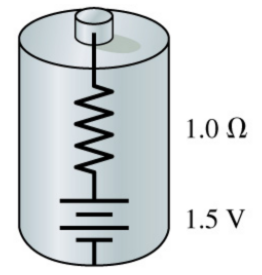# Problem: A real battery is not just an emf. We can model a real 1.5 V battery as a 1.5 V emf in series with a resistor known as the "internal resistance", as shown in the figure. A typical battery has 1.0 Ω internal resistance due to imperfections that limit current through the battery. When there's no current through the battery, and thus no voltage drop across the internal resistance, the potential difference between its terminals is 1.5 V, the value of the emf. Suppose the terminals of this battery are connected to a 2.4 Ω resistor.What fraction of the battery's power is dissipated by the internal resistance? ΔP/P= %

###### FREE Expert Solution

Power in circuits:

$\overline{){\mathbf{P}}{\mathbf{=}}{\mathbf{V}}{\mathbf{i}}{\mathbf{=}}{{\mathbf{i}}}^{{\mathbf{2}}}{\mathbf{R}}{\mathbf{=}}\frac{{\mathbf{V}}^{\mathbf{2}}}{\mathbf{R}}}$

99% (380 ratings)###### Problem Details

A real battery is not just an emf. We can model a real 1.5 V battery as a 1.5 V emf in series with a resistor known as the "internal resistance", as shown in the figure. A typical battery has 1.0 Ω internal resistance due to imperfections that limit current through the battery. When there's no current through the battery, and thus no voltage drop across the internal resistance, the potential difference between its terminals is 1.5 V, the value of the emf. Suppose the terminals of this battery are connected to a 2.4 Ω resistor.What fraction of the battery's power is dissipated by the internal resistance?

ΔP/P= %